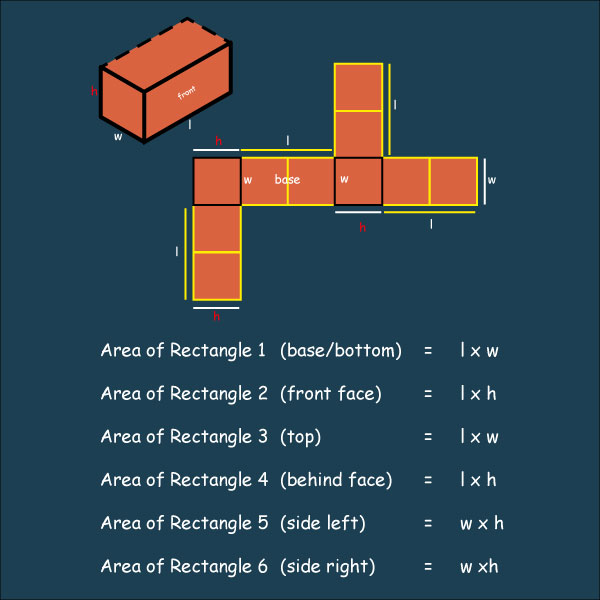Writing numbers in symbols and in words

Writing numbers in words can be done by using table like the one shown next.#### Example 1

What is 432 165 in words?

Solution

Digit 4 is found in the column of hundreds of thousands

Digit 3 in tens of thousands

Digit 2 in thousands

Digit 1 in hundreds

Digit 6 in tens

Digit 5 in ones

Therefore, the figure in words is four hundred thirty two thousand, one hundred and sixty five.

Look at the number in the table below:

 Millions Hundred Thousands Ten Thousands Thousands Hundreds Tens Ones Decimal Point Tenths Hundredth 4 3 2 1 6 5 .

#### Example 2

Write 9 300.65 in words.

Solution

Note that the digit 6 is after the decimal point and in the tenths while the digit 5 is in the hundredths position.

Therefore, we read it as point six five but when writing we write it as sixty five hundredths.

Hence 9300.65 in words is nine thousand three hundred and sixty five hundredths.

Numbers in words can also be written in figures (numerals).

Example 3

Write twenty three thousand five hundred and six in figures.

Solution

23 000 ………. twenty three thousand

+506 ………..five hundred and six

23 506 ……. twenty three thousand five hundred and six

#### Example  4

What is two million two thousand and two in numerals?

Solution

2 000 000 ………….. two million

+ 2 002 …………. two thousand and two

2 002 002 ……….. two million two thousand and two.

#### Example 5

Write four thousand two hundred three and thirty five hundredths.

Solution

Four thousand two hundred and three added to thirty five hundredths.

4 203 + 0.35 = 4 203.35